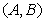## Figures index

#### Arindam Chakraborty, Anirban Ray, A. Roy Chowdhury

International Journal of Physics. 2013, 1(4), 94-100 doi:10.12691/ijp-1-4-3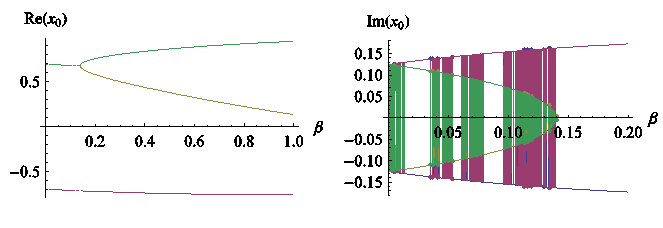• Figure 1. Variation of real and imaginary part of solution of Eq. (15)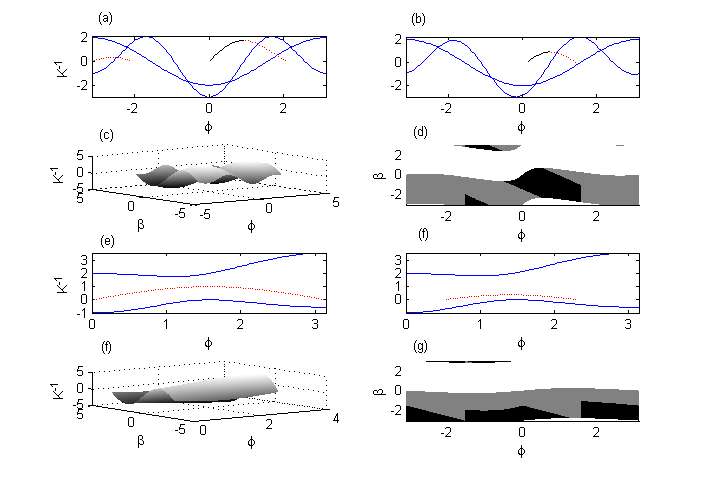• Figure 2. (a) Plot of functions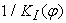where stable solutions are shown in solid black lines and the unstable solutions in dashed red lines and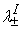(thin blue lines) for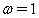and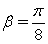. (b) Plot of functions ofwhere stable solutions are shown in solid black lines and the unstable solutions in dashed red lines and(thin blue lines) forand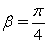. (c)Variation of functionson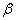vs.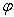plane. (d) Stable region(black) and unstable region (gray) of functionsonvs.plane. (e) Plot of functions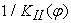where stable solutions are shown in solid black lines and the unstable solutions in dashed red lines and(thin blue lines) forand. (f) Plot of functions ofwhere stable solutions are shown in solid black lines and the unstable solutions in dashed red lines and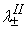(thin blue lines) forand. (g)Variation of functionsonvs.plane. (h) Stable region(black) and unstable region (gray) of functionsonvs.plane.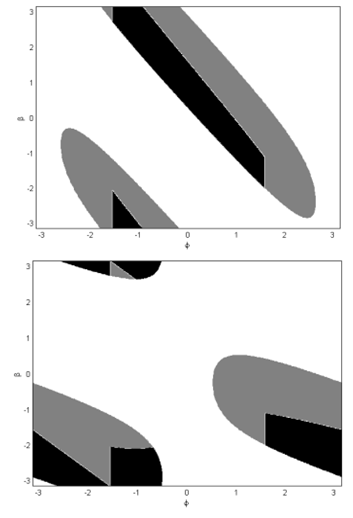• Figure 3. (a) Stable region(black) and unstable region (gray) of functions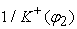onvs.plane. (b) Stable region(black) and unstable region (gray) of functions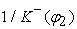onvs.plane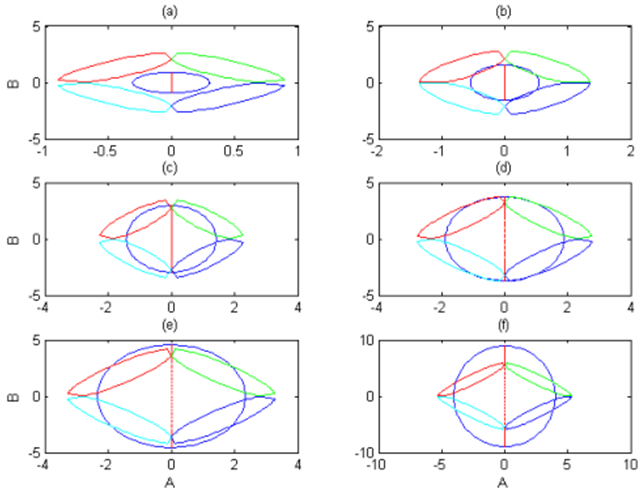• Figure 4. Variation of Eq. (33) in parameter plane